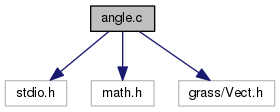GRASS Programmer's Manual  6.5.svn(2014)-r66266
angle.c File Reference
`#include <stdio.h>`
`#include <math.h>`
`#include <grass/Vect.h>`
Include dependency graph for angle.c:Go to the source code of this file.

## Functions

float dig_calc_begin_angle (struct line_pnts *points, double thresh)

float dig_calc_end_angle (struct line_pnts *points, double thresh)

int dig_is_line_degenerate (struct line_pnts *points, double thresh)

int dig_line_degenerate (struct line_pnts *points)

## Function Documentation

 float dig_calc_begin_angle ( struct line_pnts * points, double thresh )

Definition at line 31 of file angle.c.

References dig_line_degenerate().

Referenced by dig_node_add_line().

 float dig_calc_end_angle ( struct line_pnts * points, double thresh )

Definition at line 82 of file angle.c.

References dig_line_degenerate().

Referenced by dig_node_add_line().

 int dig_is_line_degenerate ( struct line_pnts * points, double thresh )

Definition at line 135 of file angle.c.

 int dig_line_degenerate ( struct line_pnts * points )

Definition at line 180 of file angle.c.

References G_debug().

Referenced by dig_calc_begin_angle(), dig_calc_end_angle(), and Vect_line_intersection().